# Algebra - math word problems

#### Number of problems found: 2906

• Rings - intersect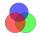There are 15 pupils on the sporting ring. 10 pupils go to football, 8 pupils go to floorball. How many pupils go to both rings at the same time?
• Balls groups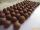Karel pulled the balls out of his pocket and divide it into the groups. He could divide them in four, six or seven, and no ball ever left. How little could be a ball?
• Savings 2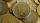Jozef and Michael saved 46 euros together. Michael saved 22 euros more than Jozef. How much did save each of them?
• Variations 4/2Determine the number of items when the count of variations of fourth class without repeating is 600 times larger than the count of variations of second class without repetition.
• Balls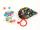Michal said to Martin: give me one ball and I'll have twice as you. Martin said: give me 4 and we will have equally. How many balls each have?
• Volume of sphereHow many times does the volume of a sphere increase if its radius increases 2 ×?
• Linear systemSolve this linear system (two linear equations with two unknowns): x+y =36 19x+22y=720
• Geometric sequence 4It is given geometric sequence a3 = 7 and a12 = 3. Calculate s23 (= sum of the first 23 members of the sequence).
• 14 yearsKája is 14 years old. Mom 44. How many years will mom be 4 times older?
• Forest nurseryIn the forest nursery after winter, they found that 1/10 stems died out of them. For them, they land 193 new spruces. How many spruces are in the forest nursery?
• Perimeter to areaCalculate the area of a circle with the perimeter 15 meters.
• Supplementary angles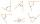One of the supplementary angles are three times larger than the other. What size is larger of supplementary angles?
• Two numbers 6Fill two natural numbers a, b: 7 + blank- blank = 5
• Chord of triangle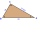If the whole chord of the triangle is 14.4 cm long, how do you calculate the shorter and longer part?
• Poplar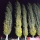How tall is a poplar by the river, if we know that 1/5 of its total height is a trunk, 1/10th of the height is the root and 35m from the trunk to the top of the poplar?
• The farmer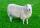The farmer had 140 sheep. For the next year, she decided to change the number of sheep in ratio 10: 7. How many sheep will he have then?
• Simple sequence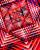Continue with this series of numbers: 1792,448, 112, _, _
• Charles Bridge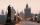Charles Bridge was 15 times older than Frantův bridge from 1900 in 1931. How old is the Charles Bridge?
• Apples 2How many apples were originally on the tree, if the first day fell one third, the second day quarter of the rest and on tree remained 45 apples?
• QuizQ2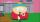The square of the first number is equal to three-fifths of the second number. Determine both numbers if you know that the second number is 5 times greater than the first, and neither of numbers is not equal to zero.

Do you have an interesting mathematical word problem that you can't solve it? Submit a math problem, and we can try to solve it.

We will send a solution to your e-mail address. Solved examples are also published here. Please enter the e-mail correctly and check whether you don't have a full mailbox.

Please do not submit problems from current active competitions such as Mathematical Olympiad, correspondence seminars etc...